### A.36 Al­ter­nate Dirac equa­tions

If you look in ad­vanced books on quan­tum me­chan­ics, you will likely find the Dirac equa­tion writ­ten in a dif­fer­ent form than given in chap­ter 12.12.

The Hamil­ton­ian eigen­value prob­lem as given in that sec­tion was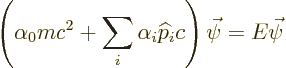where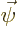was a vec­tor with four com­po­nents.

Now as­sume for a mo­ment that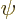is a state of def­i­nite mo­men­tum. Then the above equa­tion can be rewrit­ten in the form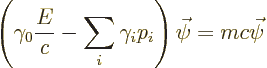The mo­ti­va­tion for do­ing so is that the co­ef­fi­cients of the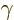ma­tri­ces are the com­po­nents of the rel­a­tivis­tic mo­men­tum four-vec­tor, chap­ter 1.3.1.

It is easy to check that the only dif­fer­ence be­tween the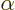andma­tri­ces is that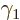through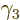get a mi­nus sign in front of their bot­tom el­e­ment. (Just mul­ti­ply the orig­i­nal equa­tion by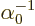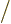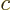and re­arrange.)

The par­en­thet­i­cal ex­pres­sion above is es­sen­tially a four-vec­tor dot prod­uct be­tween the gamma ma­tri­ces and the mo­men­tum four-vec­tor. Es­pe­cially if you give the dot prod­uct the wrong sign, as many physi­cists do. In par­tic­u­lar, in the in­dex no­ta­tion of chap­ter 1.2.5, the par­en­thet­i­cal ex­pres­sion is then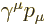. Feyn­man hit upon the bright idea to in­di­cate dot prod­ucts withma­tri­ces by a slash through the name. So you are likely to find the above equa­tion as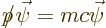Isn’t it beau­ti­fully con­cise? Isn’t it com­pletely in­com­pre­hen­si­ble?

Also con­sider the case thatis not an en­ergy and mo­men­tum eigen­func­tion. In that case, the equa­tion of in­ter­est is found from the usual quan­tum sub­sti­tu­tions that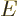be­comes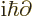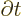and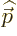be­comes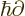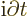. So the rewrit­ten Dirac equa­tion is then: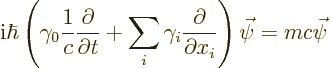In in­dex no­ta­tion, the par­en­thet­i­cal ex­pres­sion reads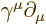. So fol­low­ing Feyn­man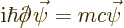Now all that the typ­i­cal physics book wants to add to that is a suit­able non-SI sys­tem of units. If you use the elec­tron mass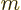as your unit of mass in­stead of the kg,as unit of ve­loc­ity in­stead of m/s, and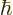as your unit of an­gu­lar mo­men­tum in­stead of kg m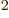/s, you get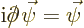No out­sider will ever guess what that stands for!